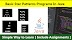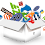FmD4FRX3FmXvDZXvGZT3FRFgNBP1w326w3z1NBMhNV5=

### Video

items# Basic Star Patterns Programs In Java

In this post, you will see some Basic Star Patterns Programs In Java. Before teaching these programs I have taught you about loops and nested loops in Java. If you don't know about loops, you can read about loops from the link given below.

In this, we have taught you how to print the Star Pattern program from Java. This will make your loops concepts stronger. And these programs are going to be very beneficial for beginners or freshers.

We are going to do all the programs dry run. So you will notice the flow of code. You need to download the dry run excel file from the link given below the program.

Three types of patterns are printed in the JAVA programming language.

1. Star Pattern
2. Number Pattern
3. Character Pattern

1. Simple Rectangle Star Pattern.

```` ````public class StarRectangle{
public static void main(String[] args){
//Outer loop for rows
for(int i=1; i<=4; i++){
//Inner loop for cols
for(int j=1; j<=4; j++){
//Print *
System.out.print("*");
}
//For new line
System.out.println();
}
}
}  ``````
``````
Output:
```` ````public class StarRectangle{
public static void main(String[] args){
//Outer loop for rows
for(int i=1; i<=4; i++){
//Inner loop for cols
for(int j=1; j<=4; j++){
//Print *
if(j%2 != 0)
System.out.print("*");
//Print =
else
System.out.print("=");
}
//For new line
System.out.println();
}
}
}  ``````
``````
Output:

#### 3. Right Angle Triangle Star Pattern.

`````` public class RightAngle{
public static void main(String[] args){
//Outer loop for rows
for(int i=1; i<=4; i++){
//Inner loop for cols
for(int j=1; j<=i; j++){
//Print *
System.out.print("*");
}
//For new line
System.out.println();
}
}
} ```` ````
``````
Output:

4. Inverted Right Angle Triangle Star Pattern.

`````` public class InvertedRightAngle{
public static void main(String[] args){
int space = 4;
//Outer loop for rows
for(int i=1; i<=4; i++){
for(int j=space; j>=i; j--){
//For Space
System.out.print(" ");
}
//Inner loop for cols
for(int k=1; k<=i; k++){
//Print *
System.out.print("*");
}
//For new line
System.out.println();
}
}
}``````
``````
Output:

Popular IT Jobs

### 5. Pyramid Star Pattern.

``````  public class InvertedRightAngle{
public static void main(String[] args){
int space = 4;
//Outer loop for rows
for(int i=1; i<=4; i++){
for(int j=space; j>=i; j--){
//For Space
System.out.print(" ");
}
//Inner loop for cols
for(int k=1; k<=i; k++){
//Print *
System.out.print("* ");
}
//For new line
System.out.println();
}
}
}````   ````

Output:

Practice well and become an expert in Java programming. With the help of these basic star pattern programs you can solve the next pattern yourself

## JAVA Star Pattern Programs Examples for Practice

Solve the following Java Star Pattern programs on your skills. And if not, the solutions will be found in the next post.

1. Sandglass Java Star Pattern Program.

``````  * * *
* *
*
*
* *
* * *``````
``````

2. Diamond Pattern Program In Java.

``````     *
***
*****
*******
*********
*******
*****
***
*  ``````
1.